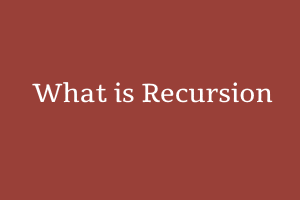Author - StudySection Post Views - 44 views# What is Recursion

## What is Recursion?

Recursion is a technique in which the resolution of one problem depends on the resolution of a smaller problem of a big problem.
With this, we divide the big problem into small problems and then solve them. To put it another way, a function calls itself in recursion to tackle smaller issue recursion. Recursion is a popular approach for solving problems because recursive solutions are generally easier to think about than their iterative counterparts and the code is also shorter and easier to understand.

### How Does Recursion Work?

Recursion is consist of three steps as follows:

1. Base Case: A recursive method must have a condition at which the method will stop calling itself. Such a condition is called the base case.
2. Recursive Call: The recursive method will recursively call itself on the smaller problem of the big problem. While writing this step, we must exercise caution because it is crucial to identify the smaller problem whose resolution the resolution of the primary problem requires.
3. Every time a recursive call is made, we typically conduct a small calculation step. Depending on the problem, we can either do this computation step before or after the recursive call.

### Example:

```#include <iostream> using namespace std; int fibo(int x) { if((x==1)||(x==0)) { return(x); }else { return(fibo(x-1)+fibo(x-2)); } } int main() { int x , i=0; cout << "Enter the number of elements : "; cin >> x; cout << "\nFibonnaci Series : "; while(i < x) { cout << " " << fibo(i); i++; } return 0; }```

Microsoft Windows 10 is a widely used operating system in computers all over the world. If you have skills in Microsoft Windows 10 then you can take our get a Windows 10 Certification from StudySection which can help you in getting hired. A beginner level certification exam for newbies and an advanced level certification exam for experts are available on StudySection.